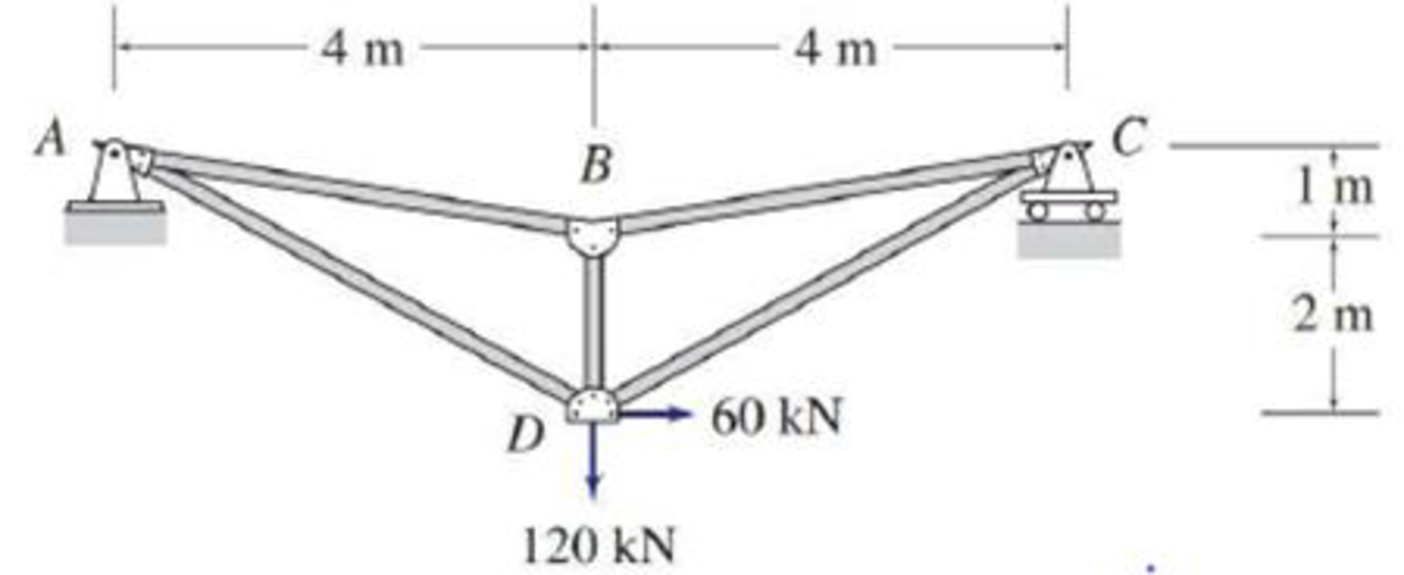# 4.6 through 4.28 Determine the force in each member of the truss shown by the method of joints. FIG. P4.7

#### Solutions

Chapter
Section
Chapter 4, Problem 7P
Textbook Problem
463 views

## 4.6 through 4.28 Determine the force in each member of the truss shown by the method of joints.FIG. P4.7

To determine

Find the forces in the members of the truss by the method of joints.

### Explanation of Solution

Given information:

Apply the sign conventions for calculating reactions, forces and moments using the three equations of equilibrium as shown below.

• For summation of forces along x-direction is equal to zero (Fx=0), consider the forces acting towards right side as positive (+) and the forces acting towards left side as negative ().
• For summation of forces along y-direction is equal to zero (Fy=0), consider the upward force as positive (+) and the downward force as negative ().
• For summation of moment about a point is equal to zero (Matapoint=0), consider the clockwise moment as negative and the counter clockwise moment as positive.

Method of joints:

The negative value of force in any member indicates compression (C) and the positive value of force in any member indicates Tension (T).

Consider the horizontal and vertical reactions at A are Ax and Ay.

Consider the vertical reaction at C is Cy.

Calculation:

Show the free body diagram of the truss as shown in Figure 1.

Refer Figure 1.

For Equilibrium of forces,

Take the sum of all horizontal forces in x direction as zero.

Fx=060+Ax=0Ax=60k

Take sum of moments about the support A as zero.

MA=0Cy×8120×4+60×3=08Cy=300Cy=37.5kN

Take the sum of all vertical forces in y direction as zero.

Fy=0Ay120+Cy=0Ay120+(37.5)=0Ay=82.5kN

Show the joint A as shown in Figure 2.

Refer Figure 2.

Calculate the value of the angle θ1 and θ2 as follows:

tanθ1=14θ1=tan1(14)θ1=14.03°

tanθ2=34θ2=tan1(34)θ2=36.86°

Take the sum of all horizontal forces in x direction as zero.

Divide both sides of the above Equation by 0.8.

Take the sum of all vertical forces in y direction as zero.

Divide both sides of the above Equation by 0.599.

Equate Equation (1) and (2).

751.2125FAB=137.7290.404FAB0.8FAB=62.7FAB=(62.70.8)FAB=78.3kNFAB=78.3kN(C)

Substitute 78.3kN for FAB in Equation (1).

Show the joint C as shown in Figure 3

### Still sussing out bartleby?

Check out a sample textbook solution.

See a sample solution

#### The Solution to Your Study Problems

Bartleby provides explanations to thousands of textbook problems written by our experts, many with advanced degrees!

Get Started

Find more solutions based on key concepts
Present Example 8.3 in Chapter 8 using the format discussed in Section 4.2. Divide the example problem into Giv...

Engineering Fundamentals: An Introduction to Engineering (MindTap Course List)

Solve Problem 8.4-7 by the ultimate strength method (use the tables in Part 8 of the Manual).

Steel Design (Activate Learning with these NEW titles from Engineering!)

Why is it necessary to have blotters on both sides of a wheel?

Precision Machining Technology (MindTap Course List)

What is a disjoint subtype? Give an example.

Database Systems: Design, Implementation, & Management

What is RFID technology? Identify three practical uses for this technology.

Principles of Information Systems (MindTap Course List)

What is a subtype discriminator? Give an example of its use.

Database Systems: Design, Implementation, & Management

5. Describe a data dictionary and list the types of information it contains.

Systems Analysis and Design (Shelly Cashman Series) (MindTap Course List)

Describe uses of interactive whiteboards and force-feedback game controllers. Define the term, tactile output.

Enhanced Discovering Computers 2017 (Shelly Cashman Series) (MindTap Course List)

If your motherboard supports ECC DDR3 memory, can you substitute non-ECC DDR3 memory?

A+ Guide to Hardware (Standalone Book) (MindTap Course List)

Describe the motion of the electrode in keyhole welding.

Welding: Principles and Applications (MindTap Course List)